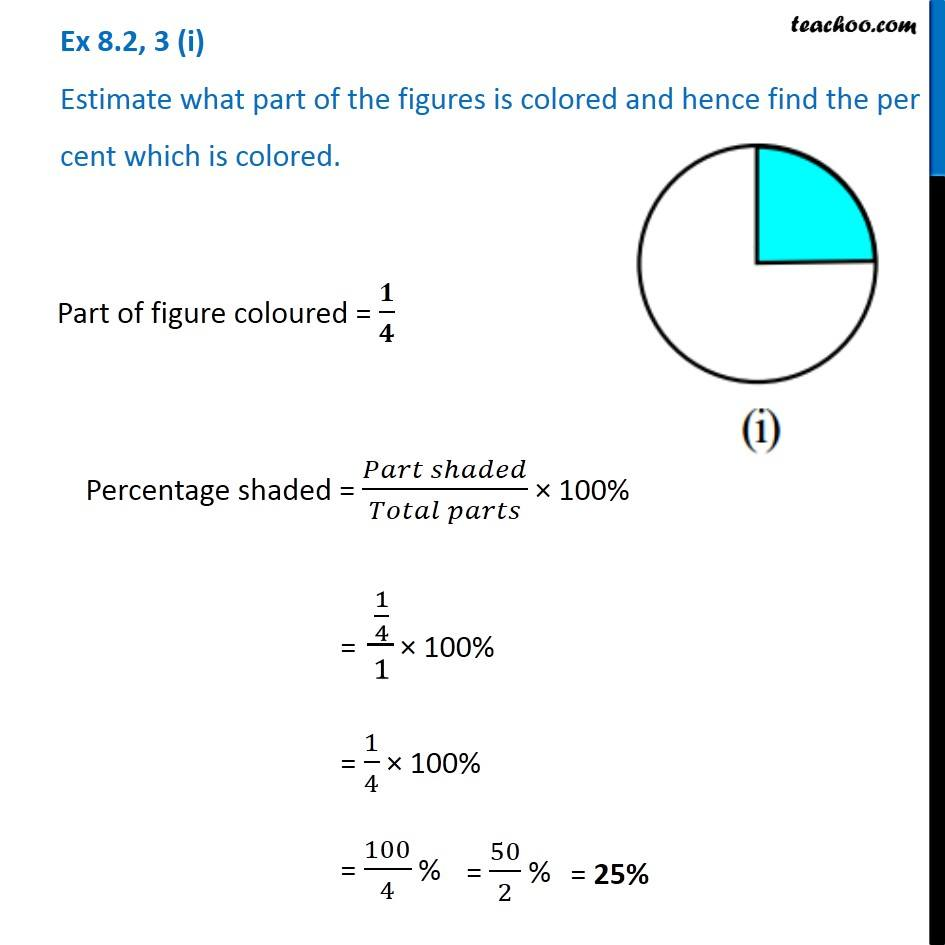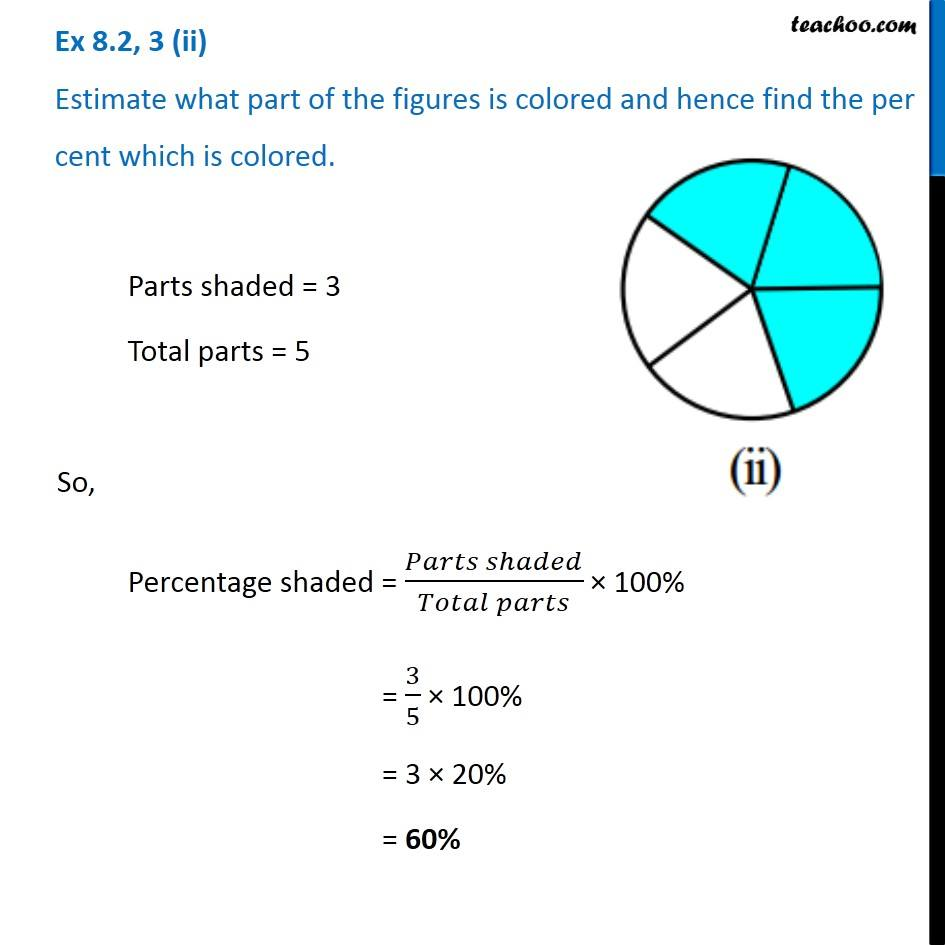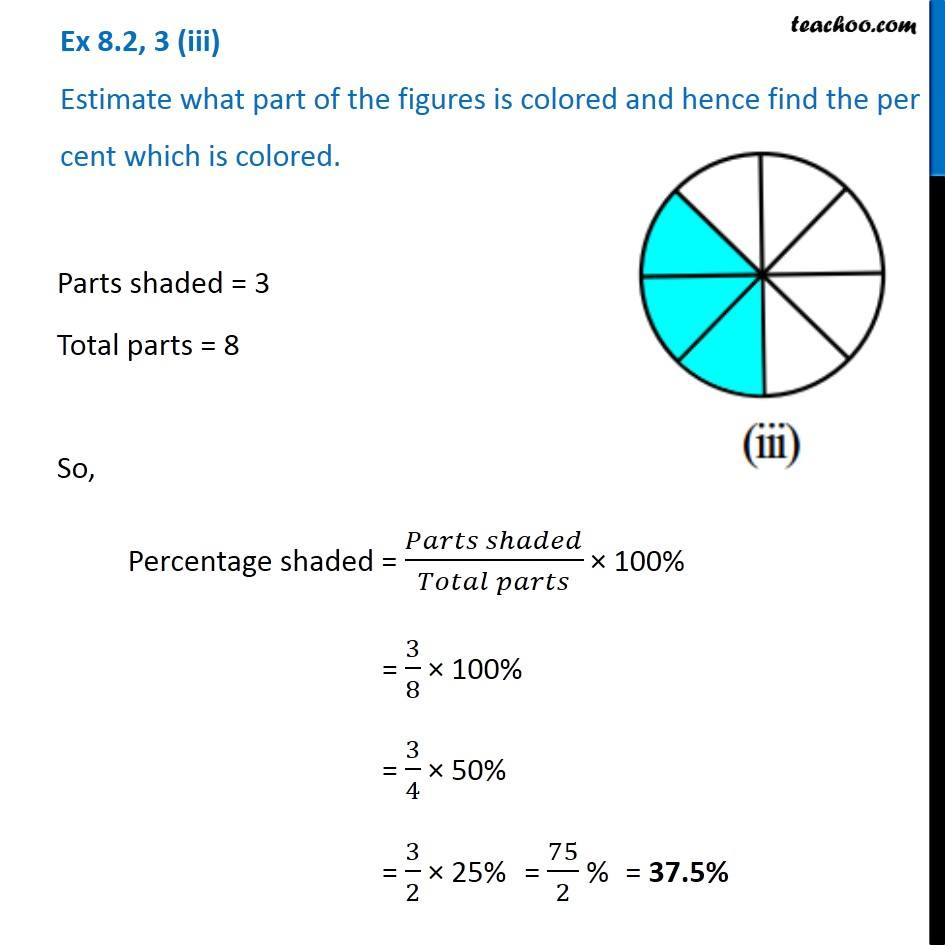Subscribe to our Youtube Channel - https://you.tube/teachoo

1. Chapter 8 Class 7 Comparing Quantities
2. Concept wise
3. Converting fraction to percent

Transcript

Ex 8.2, 3 (i) Estimate what part of the figures is colored and hence find the per cent which is colored. Part of figure coloured = 𝟏/𝟒 Percentage shaded = (𝑃𝑎𝑟𝑡 𝑠ℎ𝑎𝑑𝑒𝑑)/(𝑇𝑜𝑡𝑎𝑙 𝑝𝑎𝑟𝑡𝑠) × 100% = (1/4)/1 × 100% = 1/4 × 100% = 100/4 % Ex 8.2, 3 (ii) Estimate what part of the figures is colored and hence find the per cent which is colored. Parts shaded = 3 Total parts = 5 So, Percentage shaded = (𝑃𝑎𝑟𝑡𝑠 𝑠ℎ𝑎𝑑𝑒𝑑)/(𝑇𝑜𝑡𝑎𝑙 𝑝𝑎𝑟𝑡𝑠) × 100% = 3/5 × 100% = 3 × 20% = 60% Ex 8.2, 3 (iii) Estimate what part of the figures is colored and hence find the per cent which is colored. Parts shaded = 3 Total parts = 8 So, Percentage shaded = (𝑃𝑎𝑟𝑡𝑠 𝑠ℎ𝑎𝑑𝑒𝑑)/(𝑇𝑜𝑡𝑎𝑙 𝑝𝑎𝑟𝑡𝑠) × 100% = 3/8 × 100% = 3/4 × 50% = 3/2 × 25% = 75/2 % = 37.5%

Converting fraction to percent

About the AuthorDavneet Singh
Davneet Singh is a graduate from Indian Institute of Technology, Kanpur. He has been teaching from the past 9 years. He provides courses for Maths and Science at Teachoo.